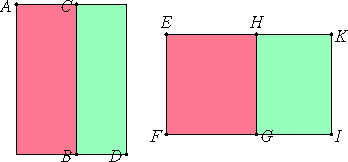# Proposition 72

If two medial areas incommensurable with one another are added together, then the remaining two irrational straight lines arise, namely either a second bimedial or a side of the sum of two medial areas.

Let two medial areas AB and CD incommensurable with one another be added together.

I say that the side of the area AD is either a second bimedial or a side of the sum of two medial areas.

For AB is either greater or less than CD.

First, let AB be greater than CD.

Set out the rational straight line EF, and apply to EF the rectangle EG equal to AB and producing EH as breadth, and the rectangle HI equal to CD and producing HK as breadth.Now, since each of the areas AB and CD is medial, therefore each of the areas EG and HI is also medial.

X.22

And they are applied to the rational straight line FE producing EH and HK as breadth, therefore each of the straight lines EH and HK is rational and incommensurable in length with EF.

Since AB is incommensurable with CD, and AB equals EG, and CD equals HI, therefore EG is also incommensurable with HI.

But EG is to HI as EH is to HK, therefore EH is incommensurable in length with HK.

X.36

Therefore EH and HK are rational straight lines commensurable in square only, therefore EK is binomial.

But the square on EH is greater than the square on HK either by the square on a straight line commensurable with EH or by the square on a straight line incommensurable with it.

First, let the square on it be greater by the square on a straight line commensurable in length with itself.

X.Def.II.3

Now neither of the straight lines EH nor HK is commensurable in length with the rational straight line EF set out, therefore EK is a third binomial.

X.56

But EF is rational, and, if an area is contained by a rational straight line and the third binomial, then the side of the area is a second bimedial, therefore the side of EI, that is, of AD, is a second bimedial.

Next, let the square on EH be greater than the square on HK by the square on a straight line incommensurable in length with EH.

X.Def.II.6

Now each of the straight lines EH and HK is incommensurable in length with EF, therefore EK is a sixth binomial.

X.59

But, if an area is contained by a rational straight line and the sixth binomial, then the side of the area is the side of the sum of two medial areas, so that the side of the area AD is also the side of the sum of two medial areas.

Therefore, if two medial areas incommensurable with one another are added together, then the remaining two irrational straight lines arise, namely either a second bimedial or a side of the sum of two medial areas.

Q.E.D.

# Proposition

The binomial straight line and the irrational straight lines after it are neither the same with the medial nor with one another.

For the square on a medial, if applied to a rational straight line, produces as breadth a straight line rational and incommensurable in length with that to which it is applied. But the square on the binomial, if applied to a rational straight line, produces as breadth the first binomial.

X.61

The square on the first bimedial, if applied to a rational straight line, produces as breadth the second binomial.

X.62

The square on the second bimedial, if applied to a rational straight line, produces as breadth the third binomial.

X.63

The square on the major, if applied to a rational straight line, produces as breadth the fourth binomial.

X.64

The square on the side of a rational plus a medial area, if applied to a rational straight line, produces as breadth the fifth binomial.

X.65

The square on the side of the sum of two medial areas, if applied to a rational straight line, produces as breadth the sixth binomial.

And the said breadths differ both from the first and from one another, from the first because it is rational, and from one another because they are not the same in order, so that the irrational straight lines themselves also differ from one another.

## Guide

This proposition is not used in the rest of the Elements